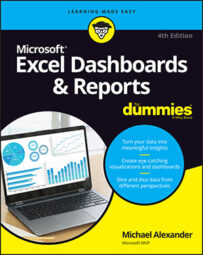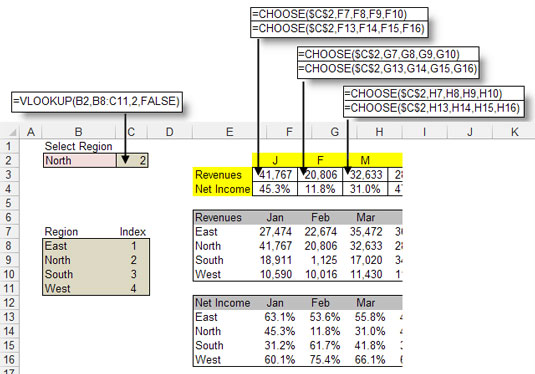##### Excel Dashboards & Reports For DummiesAlthough the CHOOSE function may not look useful on the surface, this function can dramatically enhance your Excel data models. The CHOOSE function returns a value from a specified list of values based on a specified position number.

For instance, if you enter the formulas CHOOSE(3, "Red", "Yellow", "Green", "Blue") into a cell, Excel returns Green because Green is the third item in the list of values. The formula CHOOSE(1, "Red", "Yellow", "Green", "Blue") would return Red.

## CHOOSE basics

The following figure illustrates how CHOOSE formulas can help pinpoint and extract numbers from a range of cells. Note that instead of using hard-coded values, like Red, Green, and so on, you can use cell references to list the choices.CHOOSE function allows you to find values from a defined set of choic" width="470"/>
The CHOOSE function allows you to find values from a defined set of choices.

Take a moment to review the basic syntax of the CHOOSE function:

`CHOOSE(Index_num, Value1, Value2, …)`
• Index_num: The Index_num argument specifies the position number of the chosen value in the list of values. If the third value in the list is needed, the Index_num is 3. The Index_num argument must be an integer between one and the maximum number of values in the defined list of values. That is to say, if there are ten choices defined in the CHOOSE formula, the Index_num argument can't be more than ten.

• Value: Each Value argument represents a choice in the defined list of choices for that CHOOSE formula. The Value> arguments can be hard-coded values, cell references, defined names, formulas, or functions. You can have up to 255 choices listed in your CHOOSE formulas.

## Applying CHOOSE formulas in a data model

The CHOOSE function is especially valuable in data models in which multiple layers of data need to be brought together. The following figure illustrates an example in which CHOOSE formulas help pull data together.CHOOSE formulas ensure that the appropriate data is synchronously pul" width="535"/>
The CHOOSE formulas ensure that the appropriate data is synchronously pulled from multiple data feeds.

In this example, you have two data tables: one for Revenues and one for Net Income. Each contains numbers for separate regions. The idea is to create a staging table that pulls data from both tables so that the data corresponds to a selected region.

To understand what's going on, focus on the formula in cell F3, shown in the figure. The formula is CHOOSE(\$C\$2,F7,F8,F9,F10). The Index_num argument is actually a cell reference that looks at the value in cell C2, which happens to be the number 2. As you can see, cell C2 is actually a VLOOKUP formula that pulls the appropriate index number for the selected region. The list of defined choices in the CHOOSE formula is essentially the cell references that make up the revenue values for each region: F7, F8, F9, and F10. So the formula in cell F3 translates to CHOOSE(2, 27474, 41767, 18911, 10590). The answer is 41,767.